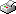Print This Page Chengcheng Hao -- doctoral thesis -- Explicit Influence Analysis in Crossover Models Abstract This dissertation develops influence diagnostics for crossover models. Mixed linear models and generalised mixed linear models are utilised to investigate continuous and count data from crossover studies, respectively. For both types of models, changes in the maximum likelihood estimates of parameters, particularly in the estimated treatment effect, due to minor perturbations of the observed data, are assessed. The novelty of this dissertation lies in the analytical derivation of influence diagnostics using decompositions of the perturbed mixed models. Consequently, the suggested influence diagnostics, referred to as the delta-beta and variance-ratio influences, provide new findings about how the constructed residuals affect the estimation in terms of different parameters of interest. The delta-beta and variance-ratio influence in three different crossover models are studied in Chapters 5-6, respectively. Chapter 5 analyses the influence of subjects in a two-period continuous crossover model. Possible problems with observation-level perturbations in crossover models are discussed. Chapter 6 extends the approach to higher-order crossover models. Furthermore, not only the individual delta-beta and variance-ratio influences of a subject are derived, but also the joint influences of two subjects from different sequences. Chapters 5-6 show that the delta-beta and variance-ratio influences of a particular parameter are decided by the special linear combination of the constructed residuals. In Chapter 7, explicit delta-beta influence on the estimated treatment effect in the two-period count crossover model is derived. The influence is related to the Pearson residuals of the subject. Graphical tools are developed to visualise information of influence concerning crossover models for both continuous and count data. Illustrative examples are provided in each chapter. Keywords: explicit maximum likelihood estimate, generalised mixed linear model, influential observation, perturbation scheme, statistical diagnostics ISBN 978-91-7649-006-8